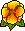### A fast semi-analytic method for the computation of elastic edge singularities

Martin Costabel, Monique Dauge, Yvon LafrancheThe singularities that we consider are the characteristic non-smooth solutions of the equations of linear elasticity in piecewise homogeneous media near two dimensional corners or three dimensional edges. We describe here a method to compute their singularity exponents and the associated angular singular functions. We present the implementation of this method in a program whose input data are geometrical data, the elasticity coefficients of each material involved and the type of boundary conditions (Dirichlet, Neumann or mixed conditions). Our method is particularly useful with anisotropic materials and allows to ``follow" the dependency of singularity exponents along a curved edge.Preprint IRMAR 98-38 (November 98)

Published in Computer Methods in Applied Mechanics and Engineering 190, 2001, 2111--2134.Fichier pdf